# MA 121 Introduction to Statistics Coaching Session 2.1.1 #19

19) Hi this is Asmita! I am a Student Success Coach and will be explaining some practice problems from the MA121 Introduction to Statistics course. Today we will review an assigned problem from Unit 2. In the “Homework Assessment” you were asked to solve this problem:

Assuming that the outcomes are equally likely, find the probability of each event in Exercise 17.

We will be using the concept of equally likely outcomes to find the probability of each outcomes.
Then we will approach this problem as follows:

1. Use the reference from exercise 17, and write down the given things.
2. Use the concept of probability of an event to find the probability of event.
3. Find the required probability by plugging the individual probabilities.

By referring Exercise 17, the sample space is
S = {bbb, bbg, bgb, bgg, gbb, gbg, ggb, ggg}.

where ‘g’ denotes girl and ‘b’ denotes boy.
“Equally likely outcomes” are the outcomes that have same probability of occurring.
Sample space S has 8 outcomes. Since the whole sample space S is an event that is certain to occur, the sum of the probabilities of all the outcomes must be 1.

Since these four outcomes are equally likely, which must add up to 1, each outcome has probability 1/8, that is,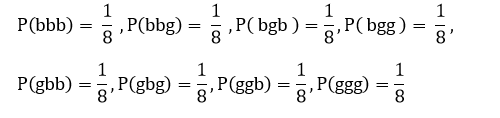a.
Step 1: Write the given things from exercise 17:
A: At least one child is a girl.
By referring Exercise 17, the outcomes for event A are
{bbg, bgb, gbb, bgg, gbg, ggb, ggg}.

Step 2: Find the probability of the event:
The probability of an event A is the sum of the probabilities of the individual outcomes in event A.
P(A) = P( bbg) + P(bgb) + P(gbb) + P(bgg) + P(gbg) + P(ggb) + P(ggg)

Step 3: Plug the individual probabilities:
There are total 7 outcomes in event A, and all are equally likely with probability 1/8.
Therefore, probability of event A is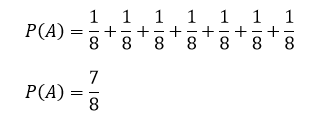b.
Step 1: Write the given things from exercise 17:
B: At most one child is a girl.
By referring Exercise 17, the outcomes for event B are
{bbb, bbg, bgb, gbb}

Step 2: Find the probability of the event:
The probability of event B is the sum of the probabilities of the individual outcomes in event B.
P(A) = [ P( bbb) + P(bbg ) + P(bgb) + P(gbb) ]

Step 3: Plug the individual probabilities:
There are total 4 outcomes in event B, and all are equally likely with probability 1/8.
Therefore, the probability of event B is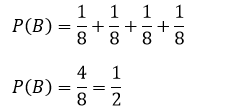c.
Step 1: Write the given things from exercise 17:
C: All of the children are girls
By referring Exercise 17, the outcome for the event C is {ggg}.

Step 2: Find the probability of the event:
The probability of event C is the sum of the probabilities of the individual outcomes in event C.
P(A) = P(ggg)

Step 3: Plug the individual probabilities:
There is only 1 outcome in the event C with probability 1/8.
Therefore, the probability of event C is
P( C ) = 1/8

d.
Step 1: Write the given things from exercise 17
Define D: Exactly two of the children are girls.
By referring Exercise 17, the outcomes for the event D are, {bgg, gbg, ggb}.

Step 2: Find the probability of the event.
The probability of event D is the sum of the probabilities of the individual outcomes in event D.
P(A) = [ P(bgg) + P(gbg) + P(ggb) ]

Step 3: Plug the individual probabilities:
There are total 3 outcomes in event D, and all are equally likely with probability 1/8.
Therefore, the probability of event D is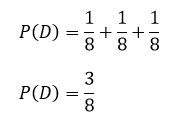e.
Step 1: Write the given things from exercise 17:
Define E: The first born is a girl.
By referring Exercise 17, the outcomes for the event E are {gbb, gbg, ggb, ggg}.

Step 2: Find the probability of the event.
The probability of event E is the sum of the probabilities of the individual outcomes in event E.
P(E) = [ P(gbb) + P(gbg) + P(ggb) + P(ggg) ]

Step 3: Plug the individual probabilities:
There are total 4 outcomes in event E, and all are equally likely with probability 1/8.
Therefore, the probability of event E is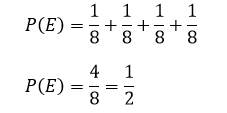Conclusion:
a. The probability of the event “At least one child is a girl” is 7/8.
b. The probability of the event “At most one child is a girl” is 1/2.
c. The probability of the event “All of the children are girls” is 1/8.
d. The probability of the event “Exactly two of the children are girls” is 3/8.
e. The probability of the event “The first born is a girl” is 1/2.

In addition to this, I am also attaching the pdf document of the practice problem for your ready reference.
Question 19.pdf (247.9 KB)

Please let me know if you have any question on this problem, or on this topic generally. I will be here in the forum for the next hour.